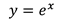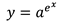Introduction

As previously noted, the Kinect sensor does not have sufficient resolution to ensure consistent accuracy of the skeleton tracking data over time. This problem manifests itself as the data seeming to vibrate around their positions. However, the Kinect SDK provides an algorithm for filtering and smoothing incoming data from the sensor, the code for which can be seen below. The smoothing algorithm parameters can be manipulated in order to provide the required level of filtering and smoothing for your desired user experience.

`            this.runtime.SkeletonEngine.TransformSmooth = true;            var parameters = new TransformSmoothParameters            {                Smoothing = 1.0f,                Correction = 0.1f,                Prediction = 0.1f,                JitterRadius = 0.05f,                MaxDeviationRadius = 0.05f            };            this.runtime.SkeletonEngine.SmoothParameters = parameters;`

A common question I’ve seen online is how does the smoothing work, and what do the various parameters do. This post attempts to answer these questions.

Smoothing and Filtering Data

Filtering is the most commonly used signal processing technique. Filters are usually used to remove or attenuate an undesired portion of a signal’s spectrum while enhancing the desired portions of the signal. The filter made available by the Kinect SDK is based on the Holt double exponential smoothing method, commonly used for statistical analysis of economic data. This algorithm provides smoothing with less latency than many other smoothing filter algorithms.

The exponential function is used to model the relationship in which a change in the independent variable, x, gives the same proportional change in the dependent variable, y.The double exponential function is a constant raised to the power of an exponential function.The simplest way to smooth a time series (data points measured at uniformly spaced time intervals) is to calculate a simple moving average, with the output being the mean of the last x data points. Exponential smoothing builds on this by assigning exponentially decreasing weights as the data points get older; one or more smoothing parameters determine the weights assigned to the data points. The smoothed data becomes a simple weighted average of the previous data point, and the previous smoothed data point. However, “good smoothing” will not be achieved until several data points have been averaged together. In addition, exponential smoothing is problematic when there is a trend in the data.

Double exponential smoothing can be defined as single exponential smoothing applied to an already exponentially smoothed time series. It performs two smoothing passes. In the first pass the smoothed data is adjusted for the trend of the previous period, by adding it to the last smoothed value. In the second pass the trend is updated, which is expressed as the difference between the last two values. Holt’s double exponential smoothing algorithm simply removes the need for a second pass of the data by smoothing the trend values directly; a number of smoothing parameters determine the weights assigned to the data points.

The algorithm parameters that the Kinect SDK allow you to set are shown in the table below, along with their default values.

 Parameter Description Default Value Comments Smoothing Specifies the amount of smoothing. 0.5 Higher values correspond to more smoothing and a value of 0 causes the raw data to be returned. Increasing smoothing tends to increase latency. Values must be in the range [0, 1.0]. Correction Specifies the amount of correction. 0.5 Lower values are slower to correct towards the raw data and appear smoother, while higher values correct toward the raw data more quickly. Values must be in the range [0, 1.0]. Prediction Specifies the number of predicted frames. 0.5 Jitter Radius Specifies the jitter-reduction radius, in meters. 0.05 The default value of 0.05 represents 5cm. Any jitter beyond the radius is clamped to the radius. Maximum Deviation Radius Specifies the maximum radius that filter positions can deviate from raw data, in meters. 0.04 Filtered values that would exceed the radius from the raw data are clamped at this distance, in the direction of the filtered value.

There is no set of “best” values to use for these parameters. Experimentation is required on an application-by-application basis in order to provide the required level of filtering and smoothing for your desired user experience.

Conclusion

The Kinect for Windows SDK beta from Microsoft Research is a starter kit for application developers. It allows access to the Kinect sensor, and experimentation with its features. The Kinect SDK provides access to Holt’s double exponential smoothing algorithm, which produces smoothing with less latency than many other smoothing filter algorithms. The algorithm parameters can be manipulated in order to provide the required level of filtering and smoothing for your desired user experience.